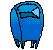Algebra Assignment #33 Graphing1. Complete the ordered pair so that each is a solution for the equation: 2x-3y = 6 (0,), ( ,0), (6, ), ( ,4) 2. Determine which of the ordered pairs are solutions for the equation 2x - 5y = 10 (5, 0), (0,2), (-5, 0), (0, -2) 3. Complete the ordered pairs so that each is a solution to the equation 3x - 2y = 30 (0, ), ( ,0), (2, ), ( , 3) 4. Complete the ordered pairs so that each is a solution to the equation 4x - y = 16 (0, ), ( , 0), ( , -4), (-2, ) 5. Complete the ordered pairs so that each is a solution to the equation 3x -4y = 12 (0, ), ( , 0), ( , 3/4), (-2/3, ) 6. Complete the ordered pairs so that each is a solution to the equation 5x - 3y = 14 (0, ), ( , 0), ( , 1/3), (4/5, )email